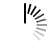# Socket Programming with Multi-threading in Python

```import socket
import sys
import traceback

def main():
start_server()

def start_server():
host = "127.0.0.1"
port = 8000        # arbitrary non-privileged port

soc = socket.socket(socket.AF_INET, socket.SOCK_STREAM)
print("Socket created")

try:
soc.bind((host, port))
except:
print("Bind failed. Error : " + str(sys.exc_info()))
sys.exit()

soc.listen(6)       # queue up to 6 requests
print("Socket now listening")

# infinite loop- do not reset for every requests
while True:
connection, address = soc.accept()
print("Connected with " + ip + ":" + port)

try:
except:
print("Thread did not start.")
traceback.print_exc()

soc.close()

def clientThread(connection, ip, port, max_buffer_size = 5120):
is_active = True

while is_active:
client_input = receive_input(connection, max_buffer_size)

if "--QUIT--" in client_input:
print("Client is requesting to quit")
connection.close()
print("Connection " + ip + ":" + port + " closed")
is_active = False
else:
print("Processed result: {}".format(client_input))
connection.sendall("-".encode("utf8"))

client_input = connection.recv(max_buffer_size)
client_input_size = sys.getsizeof(client_input)

if client_input_size > max_buffer_size:
print("The input size is greater than expected {}".format(client_input_size))

decoded_input = client_input.decode("utf8").rstrip()
result = process_input(decoded_input)

return result

def process_input(input_str):
print("Processing the input received from client")

return "Hello " + str(input_str).upper()

if __name__ == "__main__":
main()```